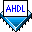## Implementing State Machines with Synchronous Outputs

 See AlsoIf the outputs of a state machine depend only on the machine's state, you can specify the state machine outputs in the `WITH STATES` clause of the State Machine Declaration. These state value assignments make state machine entry less prone to error, and in some cases, the logic may use fewer logic cells.

The moore1.tdf file shown below implements a four-state Moore state machine.

```SUBDESIGN moore1
(
clk   : INPUT;
reset : INPUT;
y     : INPUT;
z     : OUTPUT;
)
VARIABLE
%   current   current %
%   state     output  %
ss: MACHINE OF BITS (z)
WITH STATES (s0    =   0,
s1    =   1,
s2    =   1,
s3    =   0);
BEGIN
ss.clk   = clk;
ss.reset = reset;

TABLE
%  current  current     next  %
%  state    input       state %

ss,      y      =>   ss;

s0,      0      =>   s0;
s0,      1      =>   s2;
s1,      0      =>   s0;
s1,      1      =>   s2;
s2,      0      =>   s2;
s2,      1      =>   s3;
s3,      0      =>   s3;
s3,      1      =>   s1;
END TABLE;
END;
```

This example defines the states of the state machine with a State Machine Declaration. The state transitions are defined in a next-state table, which is implemented with a Truth Table Statement. In this example, machine `ss` has four states but only one state bit (`z`). The Compiler automatically adds another bit and makes appropriate assignments to this synthetic variable to produce a four-state machine. This state machine requires at least two bits.

When state values are used as outputs, as in moore1.tdf, the project may use fewer logic cells, but the logic cells may also require more logic to drive their flipflop inputs. The Compiler's Logic Synthesizer module may not be able to fully minimize the state machine in these cases.

Another way to design state machines with synchronous outputs is to omit state value assignments and to explicitly declare output flipflops. The file moore2.tdf, shown below, illustrates this alternative method.

```SUBDESIGN moore2
(
clk   : INPUT;
reset : INPUT;
y     : INPUT;
z     : OUTPUT;
)
VARIABLE
ss: MACHINE WITH STATES (s0, s1, s2, s3);
zd: NODE;
BEGIN
ss.clk   = clk;
ss.reset = reset;

z = DFF(zd, clk, VCC, VCC);

TABLE
%  current  current     next     next   %
%  state    input       state    output %
ss,      y      =>   ss,      zd;

s0,      0      =>   s0,      0;
s0,      1      =>   s2,      1;
s1,      0      =>   s0,      0;
s1,      1      =>   s2,      1;
s2,      0      =>   s2,      1;
s2,      1      =>   s3,      0;
s3,      0      =>   s3,      0;
s3,      1      =>   s1,      1;
END TABLE;
END;
```

Instead of specifying the output with state value assignments in the State Machine Declaration, this example includes a "next output" column after the "next state" column in the Truth Table Statement. This method uses a D flipflop (`DFF`) — called with an in-line logic function reference — to synchronize the outputs with the clock.